I surprised a teacher friend a month or so ago when I said how much preparation I still make before teaching a lesson, even one I’ve taught multiple times before. He was surprised. But I find it necessary to mentally walk through a lesson and have a clear plan. This is the best way I know to be prepared for what might arise and how I might deal with it. To plan for this lesson, I relied most on Chapter 11 in a book I wrote with Maryann Wickett, Teaching Arithmetic: Lessons for Extending Multiplication, Grades 4–5. Even though I’ve taught this lesson before, I took the time to reread the chapter, and then wrote a plan as a guide.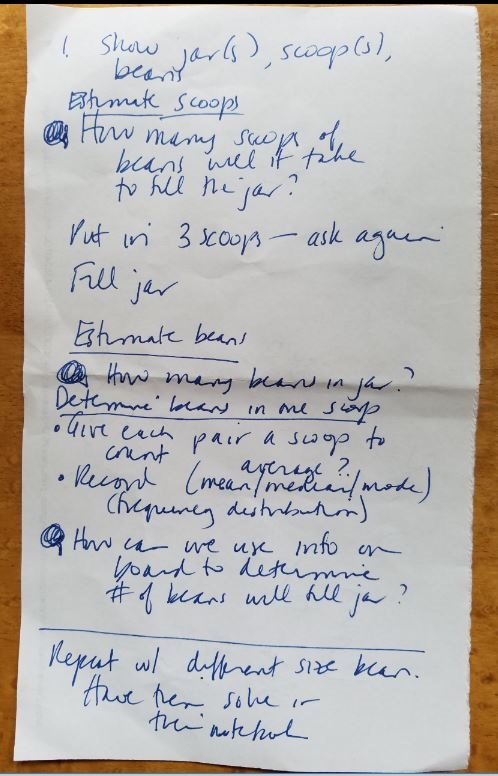My handwritten outline for the lesson indicates with colored in circles the questions I planned to ask, and includes an extension.

What the plan doesn’t describe is how I gathered the materials I needed for the lesson―beans, scoops, and jars. This took some tinkering with options of different size scoops, jars, and beans so that the lesson would engage students with appropriate numbers. (The numbers matter!) This class was comfortable with multiplying by one-digit numbers, and we were beginning to explore multiplying by two-digit numbers.

What I decided on was a scoop that held about 20 or so lima beans, and a jar that would be filled by 8 scoops. And I also had a rubber band that fit around the jar to use to indicate how full it was after several scoops.

Filling the Jar
I showed the class the scoop, beans, and jar and asked: About how many scoops of beans do you think will fill the jar? After several students gave estimates, I told them that I was going to give them a mathematical hint so that they had some information to use.

I put three scoops of beans into the jar, positioned the rubber band to show how full the jar was, held the jar up for them to see, and asked again: About how many scoops of beans do you think will fill the jar? This time I had them discuss their ideas with a partner first and then asked students to report to the class and explain their reasoning. The estimates ranged from 6 to 10.

I put three more scoops of beans in the jar, moved the rubber band, and asked again for an estimate. This narrowed the range. Finally, I filled the jar (as you can see in the photo above) to show that the jar held 8 scoops.

Presenting Another Problem
I held up the jar filled with beans and presented the next problem for them to solve: About how many beans are in the jar? Some students were willing to make a guess, but others were hesitant. I asked them if they’d like a mathematical hint, as I had given when asking them to estimate the number of scoops that filled the jar. Several asked how many beans were in a scoop.

“If you knew how many beans were in a scoop,” I asked, “how would you use that information to figure out the number of beans in the jar?” It seemed clear to them that they’d need to multiply. (No surprise, since they were in the middle of a multiplication unit.)

Deciding How Many Beans in a Scoop
I talked for a bit about how measurement was never exact, that I wasn’t sure if each time I scooped, there were exactly the same number of beans. And I explained that I would give each small group of students a scoop of beans to count. Then we’d look at the numbers and decide how many beans filled a scoop. I went around the room, distributing a scoop of beans to pairs or groups of 3. I recorded their counts on the board.

Tip #1: Practice ahead of time to be sure some scoops will be fuller than others so you get a range of numbers.
Tip #2: After recording their counts, collect the beans so that they aren’t a distraction as you move forward with the lesson.

I then used the list of numbers for an informal discussion relating to averages. With the students’ help, I reordered their counts in ascending order. I introduced the ideas of mode and median, and told them they were both ways to think about an average. The students hadn’t had experience computing means. I didn’t take the time to introduced this idea, but used “average” in a general sense.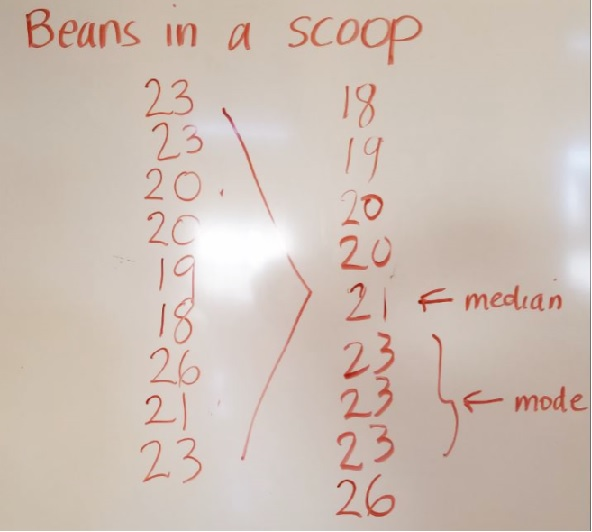With the students’ help, I reordered their counts of beans from least to greatest. Then I introduced the ideas of “median” and “mode.”

“Looking at the data on the board,” I said, “what number do you think we should use for the number of beans in a scoop?” I used the word data without defining it. I’ve found that introducing math vocabulary in the context of an experience, and using it whenever possible, is effective to help students become comfortable with new terminology.

In my handwritten plan (shown above), I had jotted down that I planned to organize the data into a frequency distribution, maybe something like this: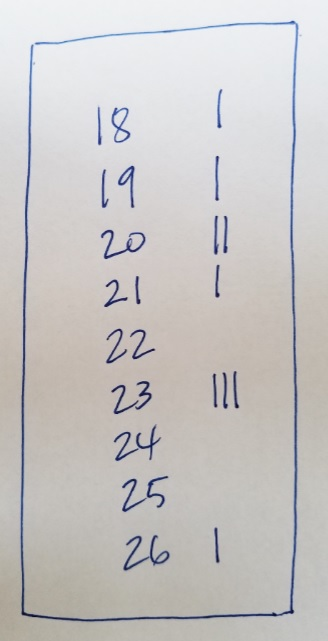I planned to display the data as a frequency distribution, but abandoned this idea to move the lesson along more quickly.

But I didn’t do this, deciding that it would be a distraction from keeping the momentum of the lesson moving toward my goal of engaging the students with multiplication. This is one of the many, many decisions we often make as we teach lessons, even those we’ve taught before.

After a short discussion, the students decided that we should use 22 as the best number for how many beans filled the scoop. When I asked why 22 since no group had counted 22 beans, they pointed out that it was between 21 and 23, the median and the mode. I didn’t pursue this further.

Figuring Out About How Many Beans Fill the Jar
The students knew that they had to multiply 8 x 22 to figure out the number of scoops in the jar. I asked them to do this in their heads and had them report the answer together, in unison. Then, as we often do in number talks, I had students present their strategies for figuring out the answer in their heads. I also offered a strategy that they hadn’t, something I do from time to time when I want to introduce an idea that didn’t emerge in the class discussion.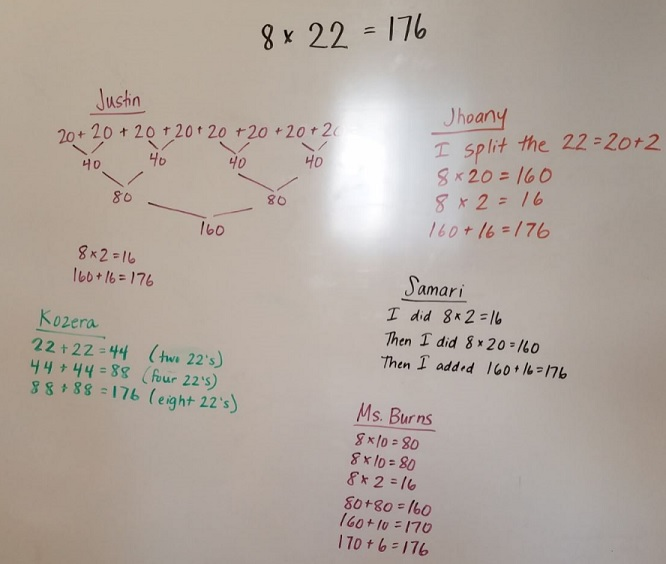The students offered different explanations for how they multiplied 8 x 22.

Extension
At this point, the time for math class was over. And, honestly, the students had done enough for one day. We returned to the lesson the next day with the same scoop but with a larger jar that held 12 scoops, giving the students the problem of multiplying 12 x 22. This problem was appropriate for this class. But instead of having a number talk as we did for 8 x 22, we asked students to solve the problem in their notebooks, working on it individually. Then students shared their methods.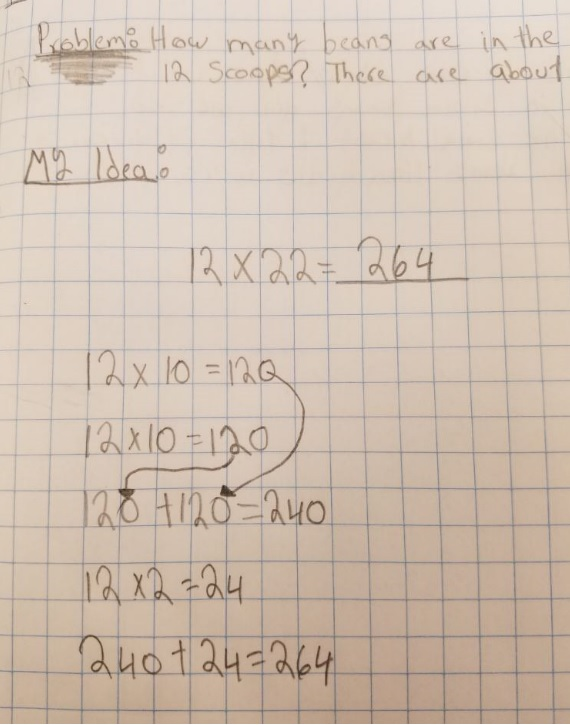This student split 22 into 10 + 10 + 2, multiplied 12 by each, and then added the partial products. His work shows his ability to apply the distributive property of multiplication over addition.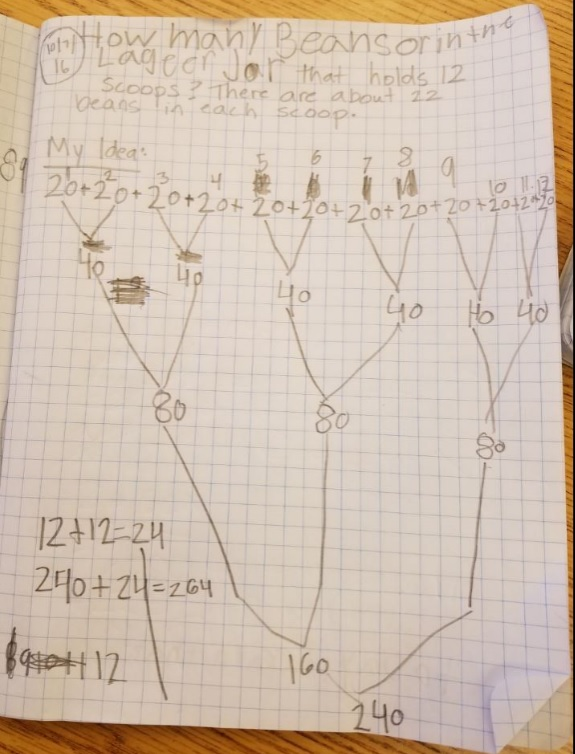This student first added 20 twelve times to get 240. Then he added 12 two times to get 264. Finally he added 240 + 24 to get 264. He worked long and hard. His work is a version of the distributive property: 12 x 22 = (12 x 20) + (12 x 2).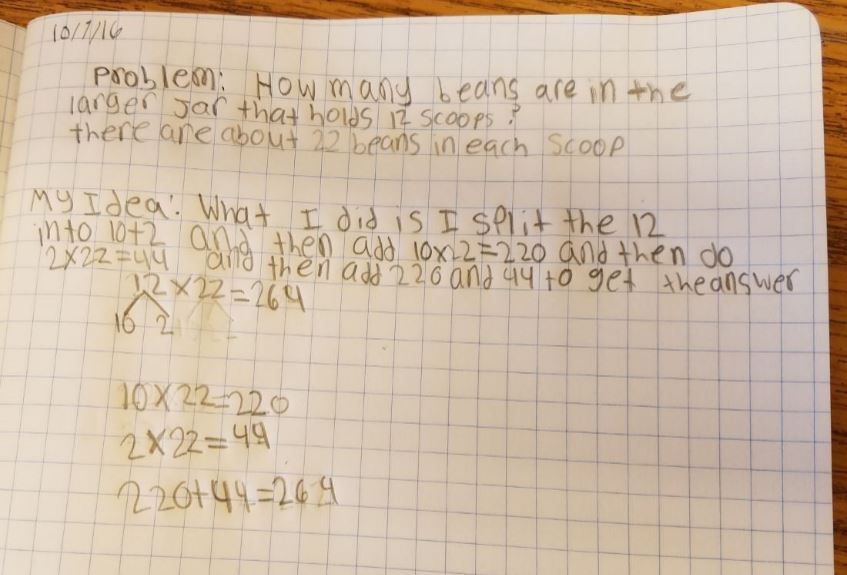This student also used the distributive property of multiplication over addition, but she split the 12 into 10 + 2, multiplied 10 and 2 each by 22, and combined the partial products.

A Different Extension
At other times, I’ve kept the same original 8-scoop jar and swapped the beans for smaller ones. This produces another problem with multiplying by 8. The students always agree that this means that there will be more beans in the jar, but it’s often a surprise to some students, and a confounding one, when they see that the jar still holds 8 scoops, even though the beans are smaller. Students often think that a different number of scoops will be needed to fill the jar, usually more scoops. This always leads to an interesting discussion.

A Final Note and a Request
Sometimes I plan a beans-and-scoops lesson for division, first counting the number of beans that fill a jar, having the class determine how many beans fill a scoop, and then figuring out how many scoops fill the jar. Then we verify their thinking by actually scooping beans to fill the jar. This lesson is in another book that Maryann Wickett and I wrote, Teaching Arithmetic: Lessons for Extending Division, Grades 4–5, Chapter 13.

With younger students, I use beans-and-scoops for place value lessons, having students count beans by 2s, 5s, and 10s to determine how many fill a scoop, and then a jar. Young children don’t always think that they’ll get the same number if they count in different ways!

If you have other ways that you’ve used beans and scoops for lessons, please post them. I’m always looking for new ideas.

### One Comment

•Brandon L says:

Hi Marilyn,

I am a candidate teacher from the Cowichan Valley in British Columbia. I want to thank you for sharing your learning about this real world math problem. I am exited to try this lesson with my class on my next practicum!